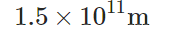# Find the magnitude of the earth's velocity. assume a year of 365 days

The radius of the earth’s very nearly circular orbit around the sun is. Find the magnitude of the earth’s velocity as it travels around the sun. Assume a year of 365 days. Express your answer using two significant figures. where v=km/s Find the magnitude of the earth’s angular velocity as it travels around the sun. Assume a year of 365 days. Express your answer using two significant figures.

where w=s−1w=s−1 Find the magnitude of the earth’s centripetal acceleration as it travels around the sun. Assume a year of 365 days. Express your answer using two significant figures. where a=m/s²